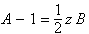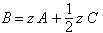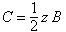Erich Prisner

## Random Walks on a path of length 4

Try the random walk on a path of length 4.

 Time:

Here the probability distributions:

 Time:

Let a(n), b(n), c(n), and d(n) denote the probabilities that the the fields are visited in the nth step. If the right field is absorbing, the recurrence formulas are

 a(n)=b(n-1)/2 b(n)=an+c(n-1)/2 c(n)=b(n)/2 d(n)=c(n)/2

with initial conditions a(0)=1, b(0)=0, c(0)=0, and d(0)=0.When solving this system, we get. The characteristic equation for the resulting recurrence relation, -4x2+3=0 has two zeros,,and, and when solving the system of linear equations we obtain, we get

d(n)=.

Obviously for even n we have d(n)=0, and for odd n we get d(n)=.

For the expected value for the transition time from the leftmost to the rightmost cell, (using a formula for a series) we getback

Erich Prisner 2004